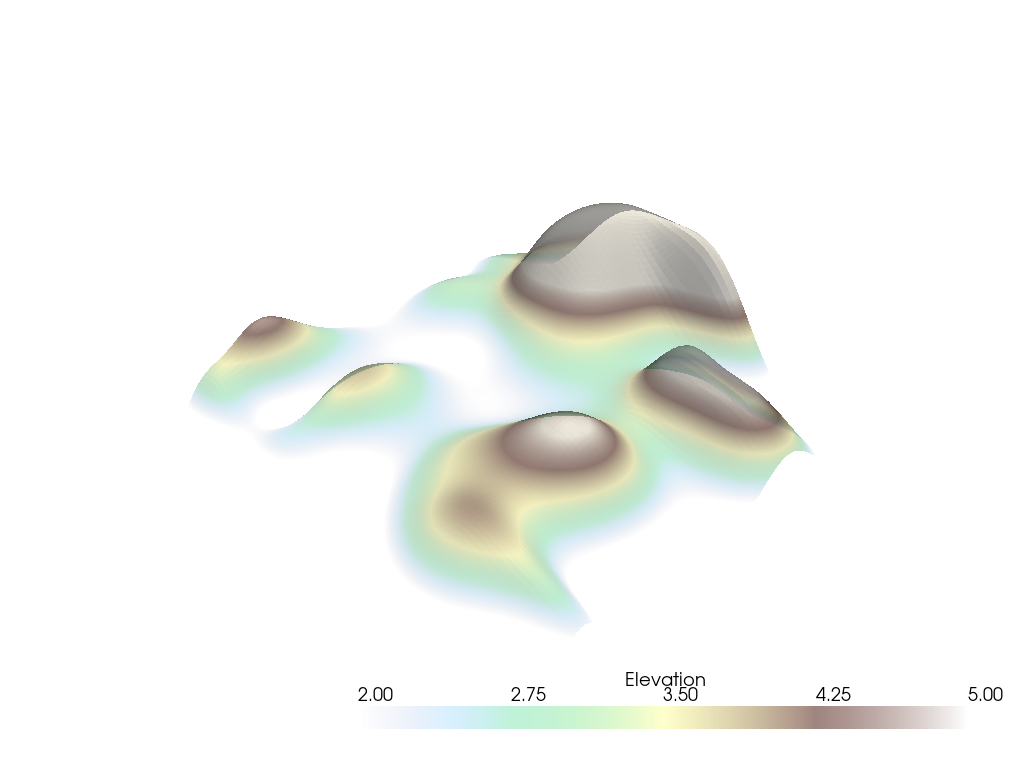# Display Options#

Take a look at the different display options offered by the `add_mesh` method.

```import pyvista as pv
from pyvista import examples

p = pv.Plotter()
p.show()
```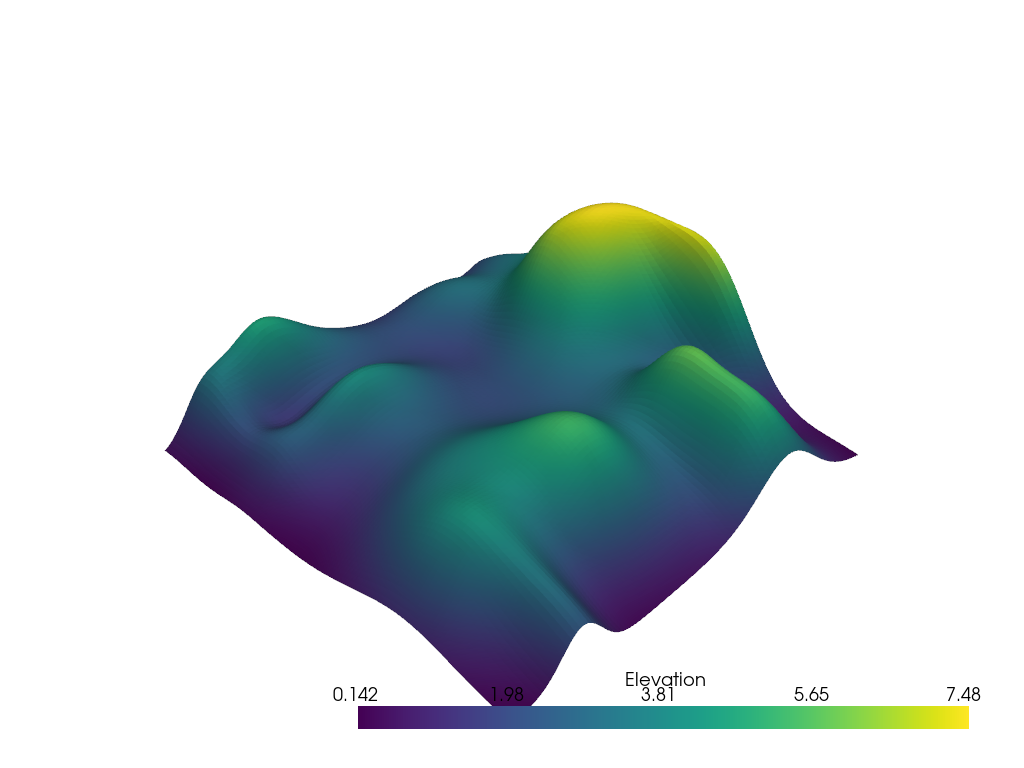Let’s take a look at some different options for the `add_mesh` method to alter how the above data are displayed.

```help(p.add_mesh)
```
```Help on method add_mesh in module pyvista.plotting.plotter:

add_mesh(mesh, color=None, style=None, scalars=None, clim=None, show_edges=None, edge_color=None, point_size=None, line_width=None, opacity=None, flip_scalars=False, lighting=None, n_colors=256, interpolate_before_map=None, cmap=None, label=None, reset_camera=None, scalar_bar_args=None, show_scalar_bar=None, multi_colors=False, name=None, texture=None, render_points_as_spheres=None, render_lines_as_tubes=None, smooth_shading=None, split_sharp_edges=None, ambient=None, diffuse=None, specular=None, specular_power=None, nan_color=None, nan_opacity=1.0, culling=None, rgb=None, categories=False, silhouette=None, use_transparency=False, below_color=None, above_color=None, annotations=None, pickable=True, preference='point', log_scale=False, pbr=None, metallic=None, roughness=None, render=True, component=None, emissive=None, copy_mesh=False, backface_params=None, show_vertices=None, **kwargs) method of pyvista.plotting.plotter.Plotter instance
Add any PyVista/VTK mesh or dataset that PyVista can wrap to the scene.

This method is using a mesh representation to view the surfaces
and/or geometry of datasets. For volume rendering, see

To see the what most of the following parameters look like in action,

Parameters
----------
mesh : pyvista.DataSet or pyvista.MultiBlock or vtk.vtkAlgorithm
Any PyVista or VTK mesh is supported. Also, any dataset
that :func:`pyvista.wrap` can handle including NumPy
arrays of XYZ points. Plotting also supports VTK algorithm
objects (``vtk.vtkAlgorithm`` and ``vtk.vtkAlgorithmOutput``).
When passing an algorithm, the rendering pipeline will be
connected to the passed algorithm to dynamically update
the scene.

color : ColorLike, optional
Use to make the entire mesh have a single solid color.
Either a string, RGB list, or hex color string.  For example:
``color='white'``, ``color='w'``, ``color=[1.0, 1.0, 1.0]``, or
``color='#FFFFFF'``. Color will be overridden if scalars are
specified.

Defaults to :attr:`pyvista.global_theme.color
<pyvista.plotting.themes.Theme.color>`.

style : str, optional
Visualization style of the mesh.  One of the following:
``style='surface'``, ``style='wireframe'``, ``style='points'``,
``style='points_gaussian'``. Defaults to ``'surface'``. Note that
``'wireframe'`` only shows a wireframe of the outer geometry.
``'points_gaussian'`` can be modified with the ``emissive``,
``render_points_as_spheres`` options.

scalars : str | numpy.ndarray, optional
Scalars used to "color" the mesh.  Accepts a string name
of an array that is present on the mesh or an array equal
to the number of cells or the number of points in the
mesh.  Array should be sized as a single vector. If both
``color`` and ``scalars`` are ``None``, then the active
scalars are used.

clim : sequence[float], optional
Two item color bar range for scalars.  Defaults to minimum and
maximum of scalars array.  Example: ``[-1, 2]``. ``rng`` is
also an accepted alias for this.

show_edges : bool, optional
Shows the edges of a mesh.  Does not apply to a wireframe
representation.

edge_color : ColorLike, optional
The solid color to give the edges when ``show_edges=True``.
Either a string, RGB list, or hex color string.

Defaults to :attr:`pyvista.global_theme.edge_color
<pyvista.plotting.themes.Theme.edge_color>`.

point_size : float, optional
Point size of any nodes in the dataset plotted. Also
applicable when style='points'. Default ``5.0``.

line_width : float, optional
Thickness of lines.  Only valid for wireframe and surface
representations.  Default ``None``.

opacity : float | str| array_like
Opacity of the mesh. If a single float value is given, it
will be the global opacity of the mesh and uniformly
applied everywhere - should be between 0 and 1. A string
can also be specified to map the scalars range to a
predefined opacity transfer function (options include:
``'linear'``, ``'linear_r'``, ``'geom'``, ``'geom_r'``).
A string could also be used to map a scalars array from
the mesh to the opacity (must have same number of elements
as the ``scalars`` argument). Or you can pass a custom
made transfer function that is an array either
``n_colors`` in length or shorter.

flip_scalars : bool, default: False
Flip direction of cmap. Most colormaps allow ``*_r``
suffix to do this as well.

lighting : bool, optional
Enable or disable view direction lighting. Default ``False``.

n_colors : int, optional
Number of colors to use when displaying scalars. Defaults to 256.
The scalar bar will also have this many colors.

interpolate_before_map : bool, optional
Enabling makes for a smoother scalars display.  Default is
``True``.  When ``False``, OpenGL will interpolate the
mapped colors which can result is showing colors that are
not present in the color map.

cmap : str | list | pyvista.LookupTable, default: :attr:`pyvista.plotting.themes.Theme.cmap`
If a string, this is the name of the ``matplotlib`` colormap to use
when mapping the ``scalars``.  See available Matplotlib colormaps.
Only applicable for when displaying ``scalars``.
``colormap`` is also an accepted alias
for this. If ``colorcet`` or ``cmocean`` are installed, their
colormaps can be specified by name.

You can also specify a list of colors to override an existing
colormap with a custom one.  For example, to create a three color
colormap you might specify ``['green', 'red', 'blue']``.

This parameter also accepts a :class:`pyvista.LookupTable`. If this
is set, all parameters controlling the color map like ``n_colors``
will be ignored.

label : str, optional
String label to use when adding a legend to the scene with

reset_camera : bool, optional
Reset the camera after adding this mesh to the scene. The default
setting is ``None``, where the camera is only reset if this plotter
has already been shown. If ``False``, the camera is not reset
regardless of the state of the ``Plotter``. When ``True``, the
camera is always reset.

scalar_bar_args : dict, optional
Dictionary of keyword arguments to pass when adding the
scalar bar to the scene. For options, see

show_scalar_bar : bool, optional
If ``False``, a scalar bar will not be added to the
scene.

multi_colors : bool, default: False
If a :class:`pyvista.MultiBlock` dataset is given this will color
each block by a solid color using matplotlib's color cycler.

name : str, optional
The name for the added mesh/actor so that it can be easily
updated.  If an actor of this name already exists in the
rendering window, it will be replaced by the new actor.

texture : pyvista.Texture or np.ndarray, optional
A texture to apply if the input mesh has texture
coordinates.  This will not work with MultiBlock
datasets.

render_points_as_spheres : bool, optional
Render points as spheres rather than dots.

render_lines_as_tubes : bool, optional
Show lines as thick tubes rather than flat lines.  Control
the width with ``line_width``.

Enable smooth shading when ``True`` using the Phong
Automatically enabled when ``pbr=True``.  See

split_sharp_edges : bool, optional
Split sharp edges exceeding 30 degrees when plotting with smooth
shading.  Control the angle with the optional keyword argument
``feature_angle``.  By default this is ``False`` unless overridden
by the global or plotter theme.  Note that enabling this will
create a copy of the input mesh within the plotter.  See

ambient : float, optional
When lighting is enabled, this is the amount of light in
the range of 0 to 1 (default 0.0) that reaches the actor
when not directed at the light source emitted from the
viewer.

diffuse : float, optional
The diffuse lighting coefficient. Default 1.0.

specular : float, optional
The specular lighting coefficient. Default 0.0.

specular_power : float, optional
The specular power. Between 0.0 and 128.0.

nan_color : ColorLike, optional
The color to use for all ``NaN`` values in the plotted
scalar array.

nan_opacity : float, optional
Opacity of ``NaN`` values.  Should be between 0 and 1.
Default 1.0.

culling : str, optional
Does not render faces that are culled. Options are
``'front'`` or ``'back'``. This can be helpful for dense
surface meshes, especially when edges are visible, but can
cause flat meshes to be partially displayed.  Defaults to
``False``.

rgb : bool, optional
If an 2 dimensional array is passed as the scalars, plot
those values as RGB(A) colors. ``rgba`` is also an
accepted alias for this.  Opacity (the A) is optional.  If
a scalars array ending with ``"_rgba"`` is passed, the default
becomes ``True``.  This can be overridden by setting this
parameter to ``False``.

categories : bool, optional
If set to ``True``, then the number of unique values in
the scalar array will be used as the ``n_colors``
argument.

silhouette : dict, bool, optional
If set to ``True``, plot a silhouette highlight for the
mesh. This feature is only available for a triangulated
``PolyData``.  As a ``dict``, it contains the properties
of the silhouette to display:

* ``color``: ``ColorLike``, color of the silhouette
* ``line_width``: ``float``, edge width
* ``opacity``: ``float`` between 0 and 1, edge transparency
* ``feature_angle``: If a ``float``, display sharp edges
exceeding that angle in degrees.
* ``decimate``: ``float`` between 0 and 1, level of decimation

use_transparency : bool, optional
Invert the opacity mappings and make the values correspond
to transparency.

below_color : ColorLike, optional
Solid color for values below the scalars range
(``clim``). This will automatically set the scalar bar
``below_label`` to ``'below'``.

above_color : ColorLike, optional
Solid color for values below the scalars range
(``clim``). This will automatically set the scalar bar
``above_label`` to ``'above'``.

annotations : dict, optional
Pass a dictionary of annotations. Keys are the float
values in the scalars range to annotate on the scalar bar
and the values are the string annotations.

pickable : bool, optional
Set whether this actor is pickable.

preference : str, default: "point"
When ``mesh.n_points == mesh.n_cells`` and setting
scalars, this parameter sets how the scalars will be
mapped to the mesh.  Default ``'point'``, causes the
scalars will be associated with the mesh points.  Can be
either ``'point'`` or ``'cell'``.

log_scale : bool, default: False
Use log scale when mapping data to colors. Scalars less
than zero are mapped to the smallest representable
positive float.

pbr : bool, optional
Enable physics based rendering (PBR) if the mesh is
``PolyData``.  Use the ``color`` argument to set the base
color.

metallic : float, optional
Usually this value is either 0 or 1 for a real material
but any value in between is valid. This parameter is only
used by PBR interpolation.

roughness : float, optional
This value has to be between 0 (glossy) and 1 (rough). A
glossy material has reflections and a high specular
part. This parameter is only used by PBR
interpolation.

render : bool, default: True
Force a render when ``True``.

component : int, optional
Set component of vector valued scalars to plot.  Must be
nonnegative, if supplied. If ``None``, the magnitude of
the vector is plotted.

emissive : bool, optional
Treat the points/splats as emissive light sources. Only valid for
``style='points_gaussian'`` representation.

copy_mesh : bool, default: False
If ``True``, a copy of the mesh will be made before adding it to
the plotter.  This is useful if you would like to add the same
mesh to a plotter multiple times and display different
scalars. Setting ``copy_mesh`` to ``False`` is necessary if you
would like to update the mesh after adding it to the plotter and
have these updates rendered, e.g. by changing the active scalars or
through an interactive widget. This should only be set to ``True``
with caution. Defaults to ``False``. This is ignored if the input
is a ``vtkAlgorithm`` subclass.

backface_params : dict | pyvista.Property, optional
A :class:`pyvista.Property` or a dict of parameters to use for
backface rendering. This is useful for instance when the inside of
oriented surfaces has a different color than the outside. When a
:class:`pyvista.Property`, this is directly used for backface
rendering. When a dict, valid keys are :class:`pyvista.Property`
attributes, and values are corresponding values to use for the
given property. Omitted keys (or the default of
``backface_params=None``) default to the corresponding frontface
properties.

show_vertices : bool, optional
When ``style`` is not ``'points'``, render the external surface
vertices. The following optional keyword arguments may be used to
control the style of the vertices:

* ``vertex_color`` - The color of the vertices
* ``vertex_style`` - Change style to ``'points_gaussian'``
* ``vertex_opacity`` - Control the opacity of the vertices

**kwargs : dict, optional
Optional keyword arguments.

Returns
-------
pyvista.plotting.actor.Actor
Actor of the mesh.

Examples
--------
Add a sphere to the plotter and show it with a custom scalar
bar title.

>>> import pyvista as pv
>>> sphere = pv.Sphere()
>>> sphere['Data'] = sphere.points[:, 2]
>>> plotter = pv.Plotter()
...     sphere, scalar_bar_args={'title': 'Z Position'}
... )
>>> plotter.show()

Plot using RGB on a single cell.  Note that since the number of
points and the number of cells are identical, we have to pass
``preference='cell'``.

>>> import pyvista as pv
>>> import numpy as np
>>> vertices = np.array(
...     [
...         [0, 0, 0],
...         [1, 0, 0],
...         [0.5, 0.667, 0],
...         [0.5, 0.33, 0.667],
...     ]
... )
>>> faces = np.hstack(
...     [[3, 0, 1, 2], [3, 0, 3, 2], [3, 0, 1, 3], [3, 1, 2, 3]]
... )
>>> mesh = pv.PolyData(vertices, faces)
>>> mesh.cell_data['colors'] = [
...     [255, 255, 255],
...     [0, 255, 0],
...     [0, 0, 255],
...     [255, 0, 0],
... ]
>>> plotter = pv.Plotter()
...     mesh,
...     scalars='colors',
...     lighting=False,
...     rgb=True,
...     preference='cell',
... )
>>> plotter.camera_position = 'xy'
>>> plotter.show()

Note how this varies from ``preference=='point'``.  This is
because each point is now being individually colored, versus
in ``preference=='point'``, each cell face is individually
colored.

>>> plotter = pv.Plotter()
...     mesh,
...     scalars='colors',
...     lighting=False,
...     rgb=True,
...     preference='point',
... )
>>> plotter.camera_position = 'xy'
>>> plotter.show()

Plot a plane with a constant color and vary its opacity by point.

>>> plane = pv.Plane()
>>> plane.plot(
...     color='b',
...     opacity=np.linspace(0, 1, plane.n_points),
...     show_edges=True,
... )

Plot the points of a sphere with Gaussian smoothing while coloring by z
position.

>>> mesh = pv.Sphere()
>>> mesh.plot(
...     scalars=mesh.points[:, 2],
...     style='points_gaussian',
...     opacity=0.5,
...     point_size=10,
...     render_points_as_spheres=False,
...     show_scalar_bar=False,
... )
```

Plot that mesh with the edges of cells displayed

```p = pv.Plotter()
p.show()
```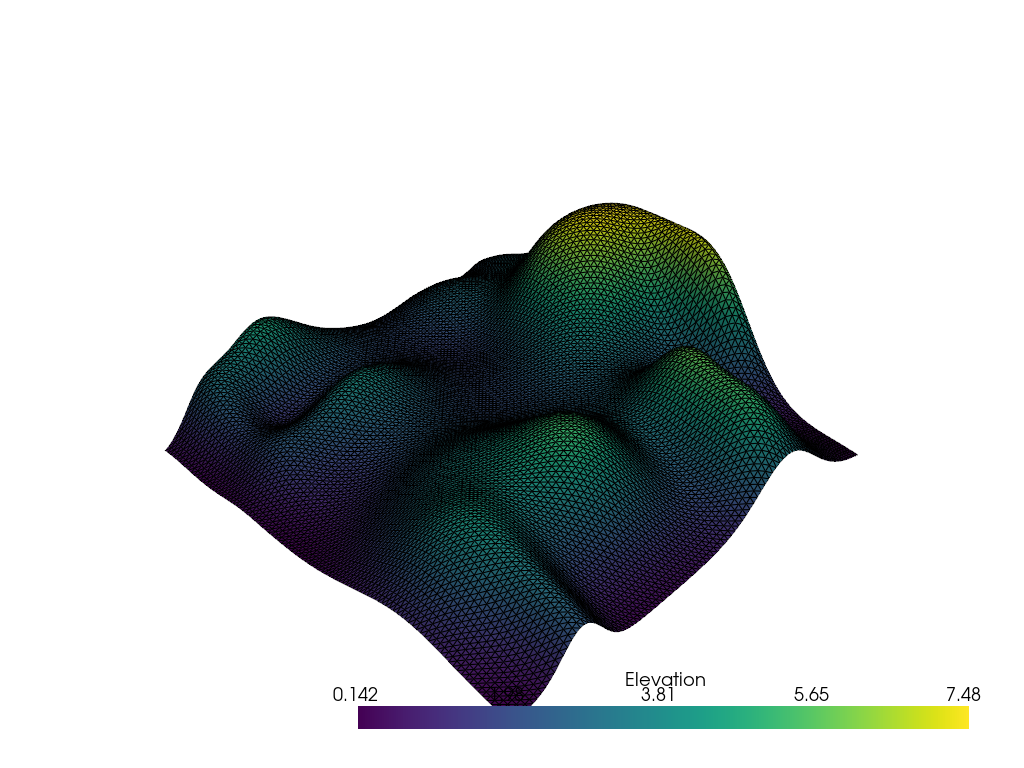Plot that mesh with the colored edges and as a show the surface as a solid color (use a named color!)

```p = pv.Plotter()
p.show()
```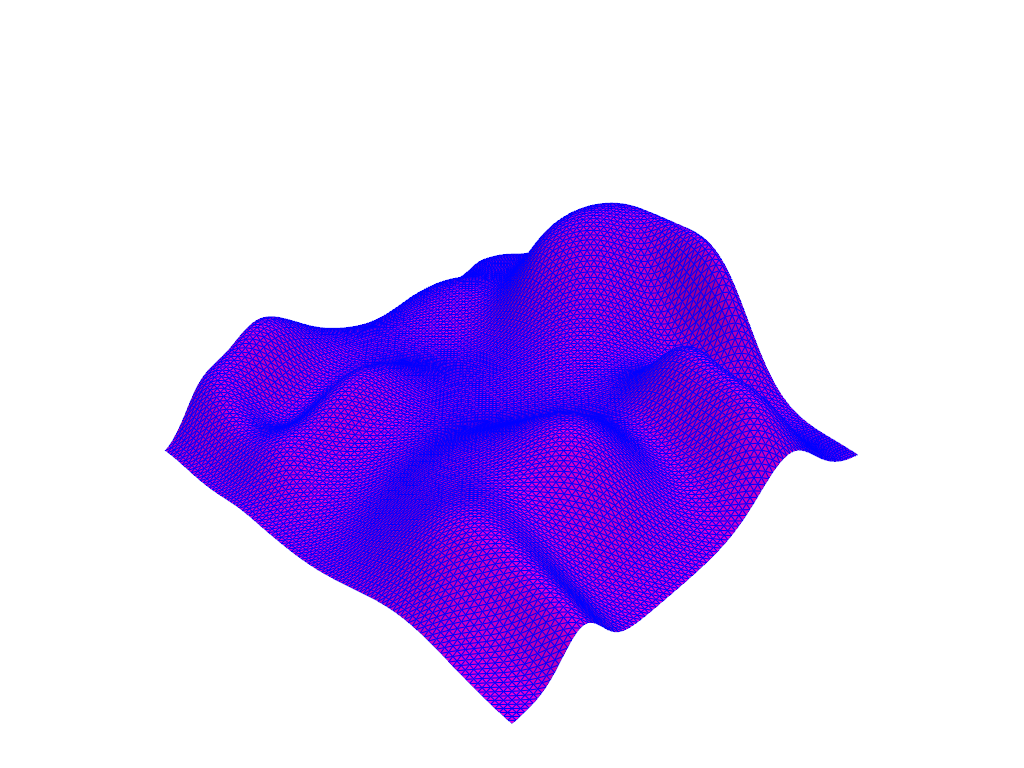Display with a points representation style

```p = pv.Plotter()
p.show()
```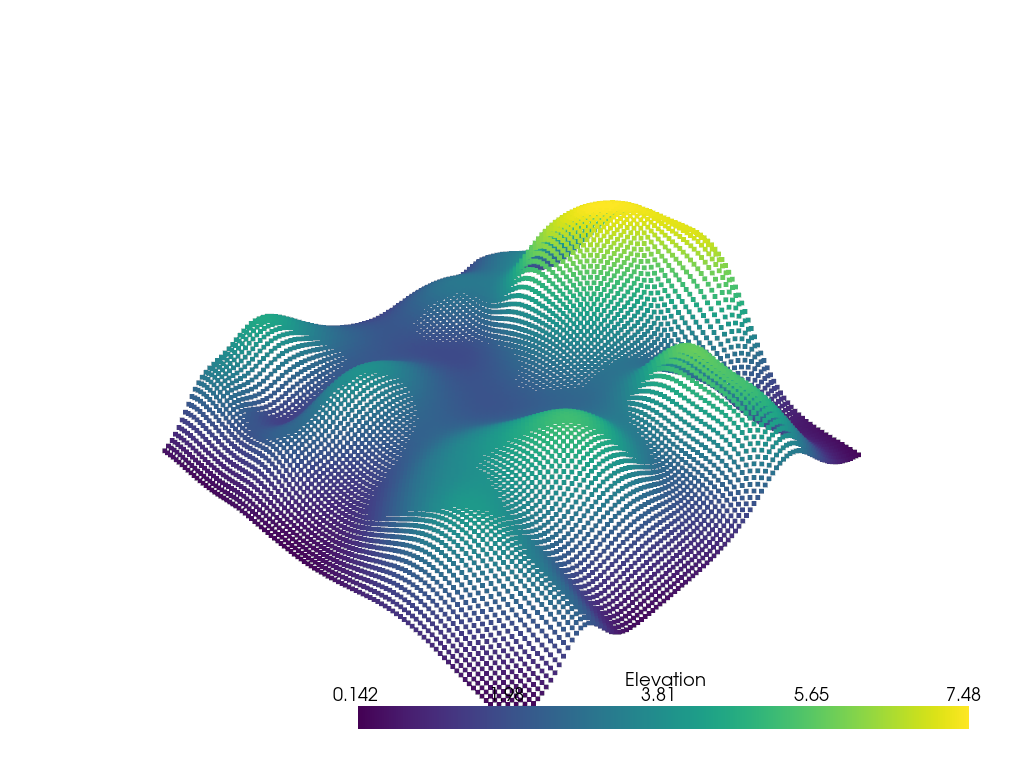And adjust the points display size

```p = pv.Plotter()
p.show()
```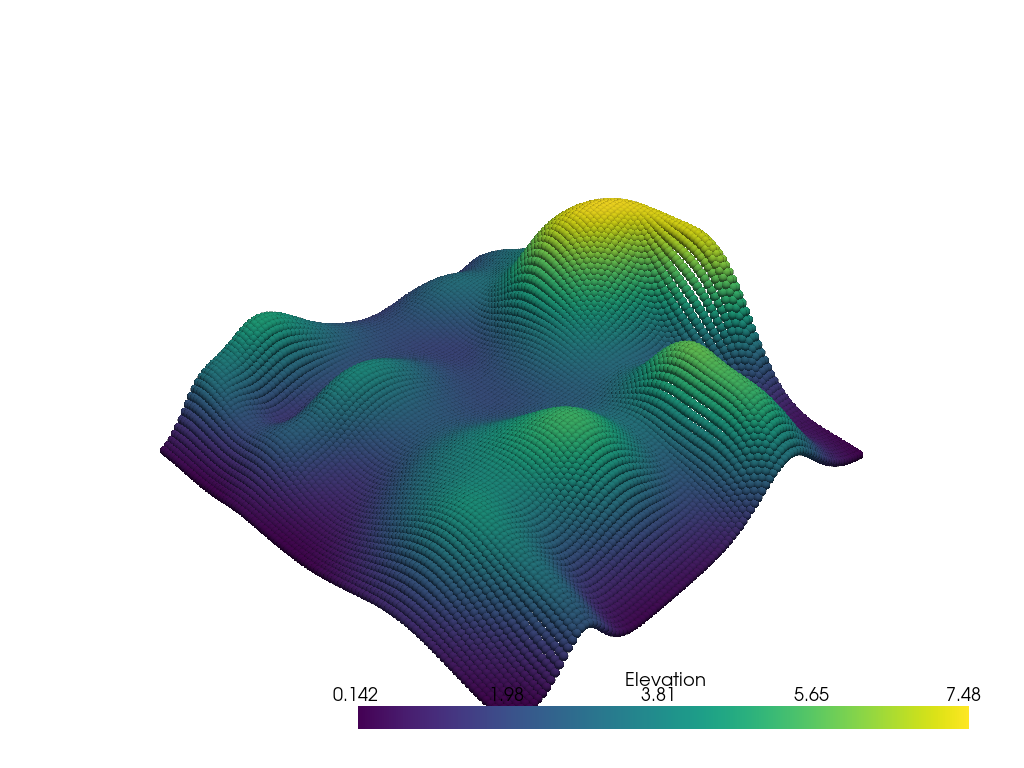Change the color map and the color limits

```p = pv.Plotter()
p.show()
```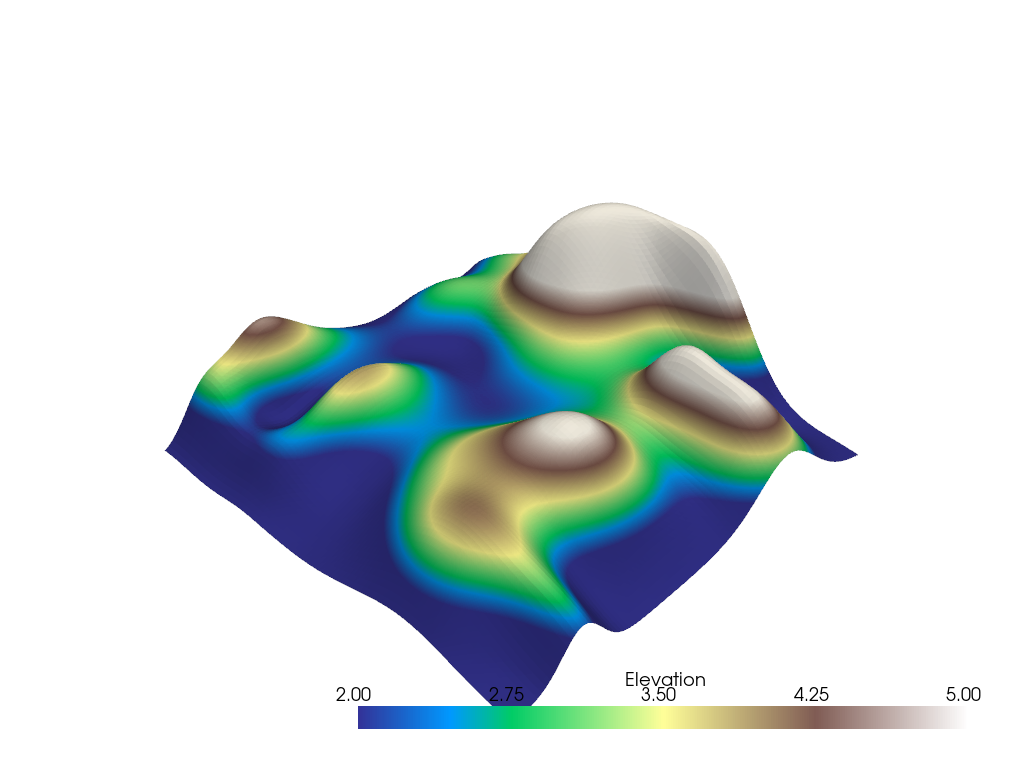```p = pv.Plotter()